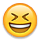Welcome to the Parallax Discussion Forums, sign-up to participate.

# Ping))) Ultrasonic Sensor

Hi,

I am using the Parallax Ping))) Ultrasonic Sensor on my Raspberry Pi 4 Model B. Maybe this could be a relevant information. I first looked at this code. But it doesn`t work. I don`t get any result. After that I looked how SunFounder uses a Ping))) ultrasonic sensor in there PiCar-S, like here.

The error that I got is:
False
False
False
False
False
....

I wired it to 5V, GND and Pin 16 which means GPIO 23 or BCM 23.

Off course I also used Python because this is a Raspberry PiHere my test_parallax.py
Spoiler:
import parallax7
import time

ultrasonic = parallax7.Parallax(23)
threshold = 10

def main():
distance = ultrasonic.get_distance()
status = ultrasonic.less_than(threshold)
if distance != -1:
print('distance', distance, 'cm')
time.sleep(0.2)
else:
print(False)
if status == 1:
print("Less than %d" % threshold)
elif status == 0:
print("Over %d" % threshold)
else:

if __name__=='__main__':
while True:
main()

This file delivers me the error code "Read distance error." which means that I can`t get any status from my other class file and the "False"-Error, which means that I can`t get any measurements. My Parallax class is inside the parallax7.py file because I tested a lot...
Spoiler:
import RPi.GPIO as GPIO
import time

class Parallax(object):
timeout = 0.05

def __init__(self, pin):
self.pin = pin
GPIO.setmode(GPIO.BCM)

def distance(self):
pulse_end = 0
pulse_start = 0

GPIO.setup(self.pin, GPIO.OUT) # Set to low
GPIO.output(self.pin, False)

time.sleep(0.01)

GPIO.output(self.pin, True) # Set high

time.sleep(0.00001)

GPIO.output(self.pin, False) # Set low
GPIO.setup(self.pin, GPIO.IN) # Set to input

timeout_start = time.time()

# Count microseconds that SIG was high
while GPIO.input(self.pin) == 0:
pulse_start = time.time()

if pulse_start - timeout_start > self.timeout:
return -1

while GPIO.input(self.pin) == 1:
pulse_end = time.time()

if pulse_start - timeout_start > self.timeout:
return -1

if pulse_start != 0 and pulse_end != 0:
pulse_duration = pulse_end - pulse_start
# The speed of sound is 340 m/s or 29 microseconds per centimeter.
# The ping travels out and back, so to find the distance of the
# object we take half of the distance travelled.
# distance = duration / 29 / 2
distance = pulse_duration * 100 * 343.0 / 2
distance = int(distance)

#print('start = %s'%pulse_start,)
#print('end = %s'%pulse_end)
if distance >= 0:
return distance
else:
return -1
else :
#print('start = %s'%pulse_start,)
#print('end = %s'%pulse_end)
return -1

def get_distance(self, mount = 5):
sum = 0

for i in range(mount):
a = self.distance()
sum += a

return int(sum/mount)

def less_than(self, alarm_gate):
dis = self.get_distance()
status = 0

if dis >= 0 and dis <= alarm_gate:
status = 1
elif dis > alarm_gate:
status = 0
else:
status = -1

return status

As you can see it`s the same code as the code from SunFounder. I am sure that it`s not a problem with the program code. Because other codes also doesn`t work.

What causes the error? Is it impossible to use a ping sensor with the Raspberry Pi?!? I am sure that the problem is that I only get the pulse_start but not the pulse_end. Could it be that the Raspberry Pi can`t "switch" from Trigger to Echo?

Hopefully we can find a good solution for this problem. Thank you very much.